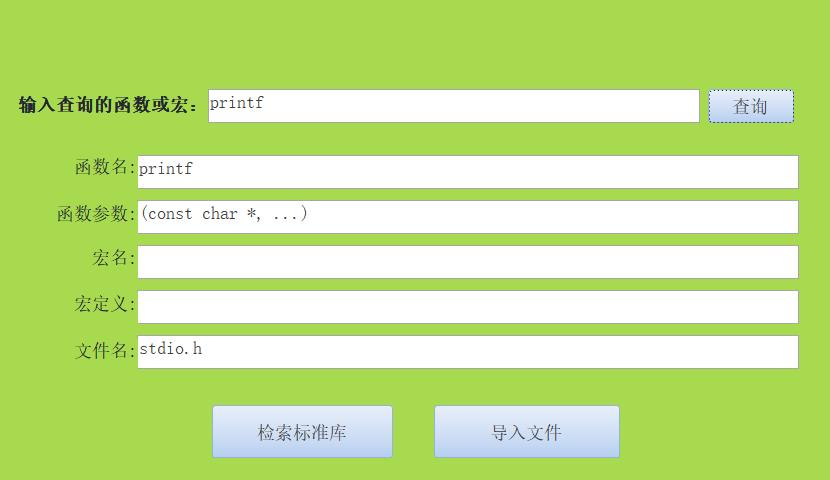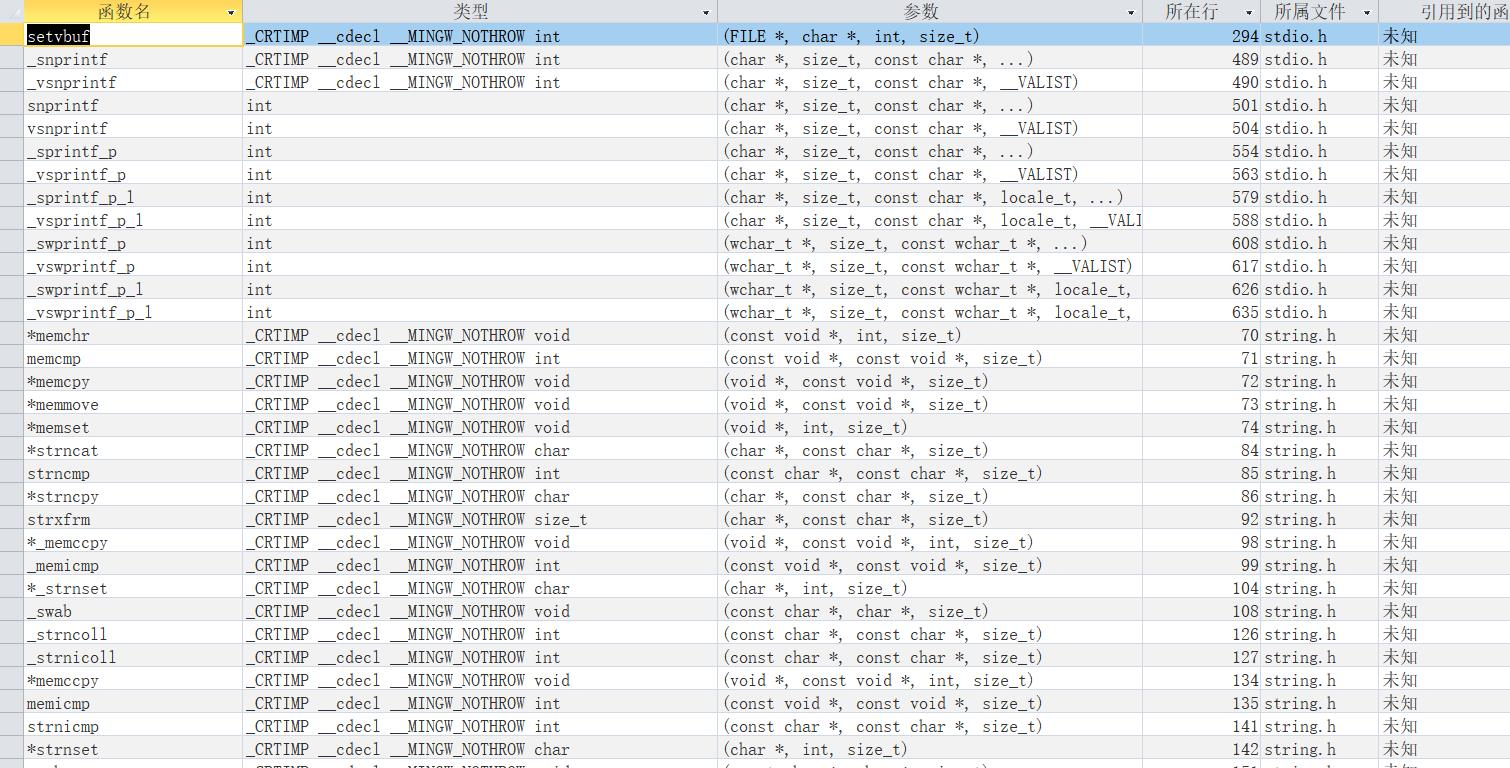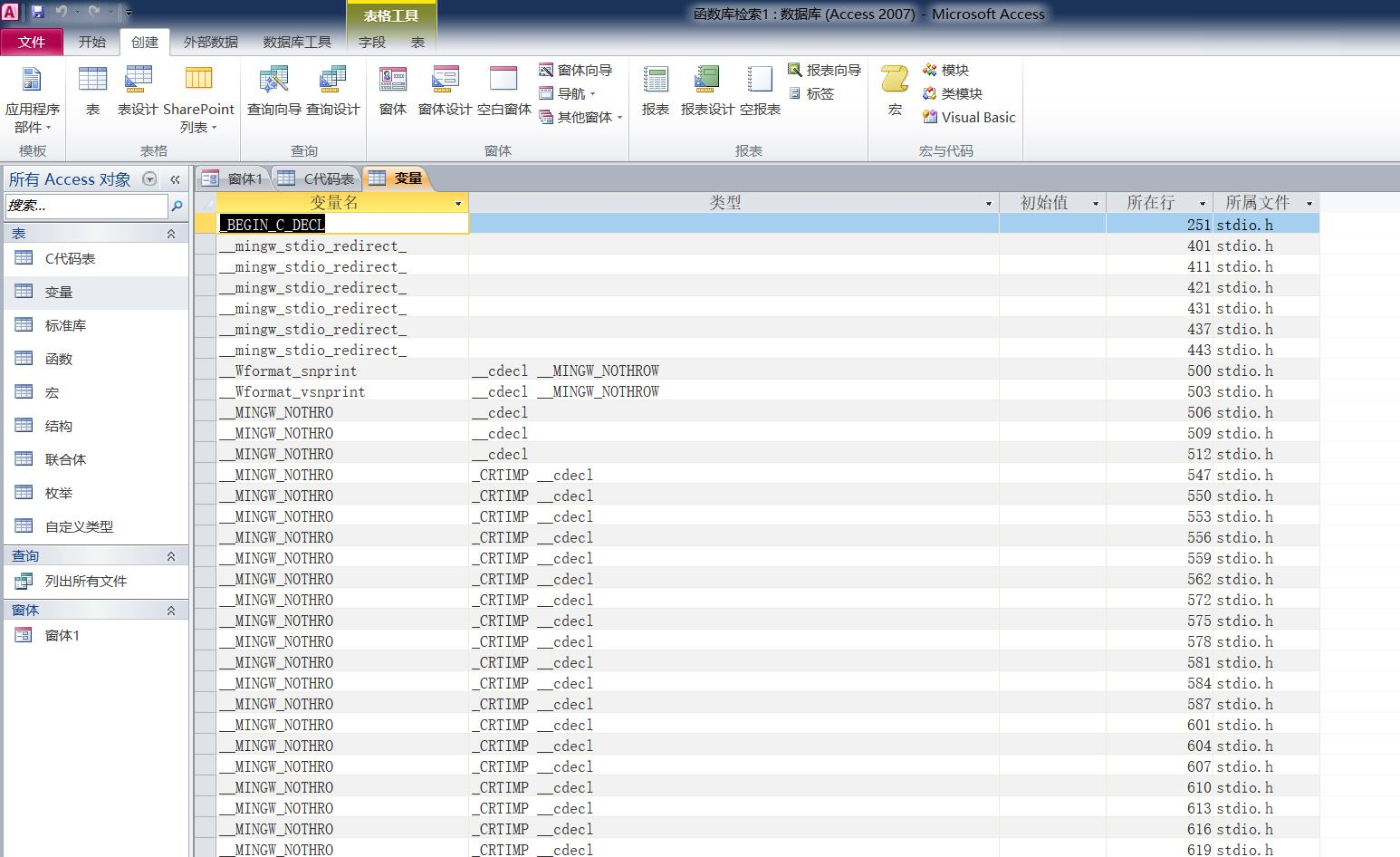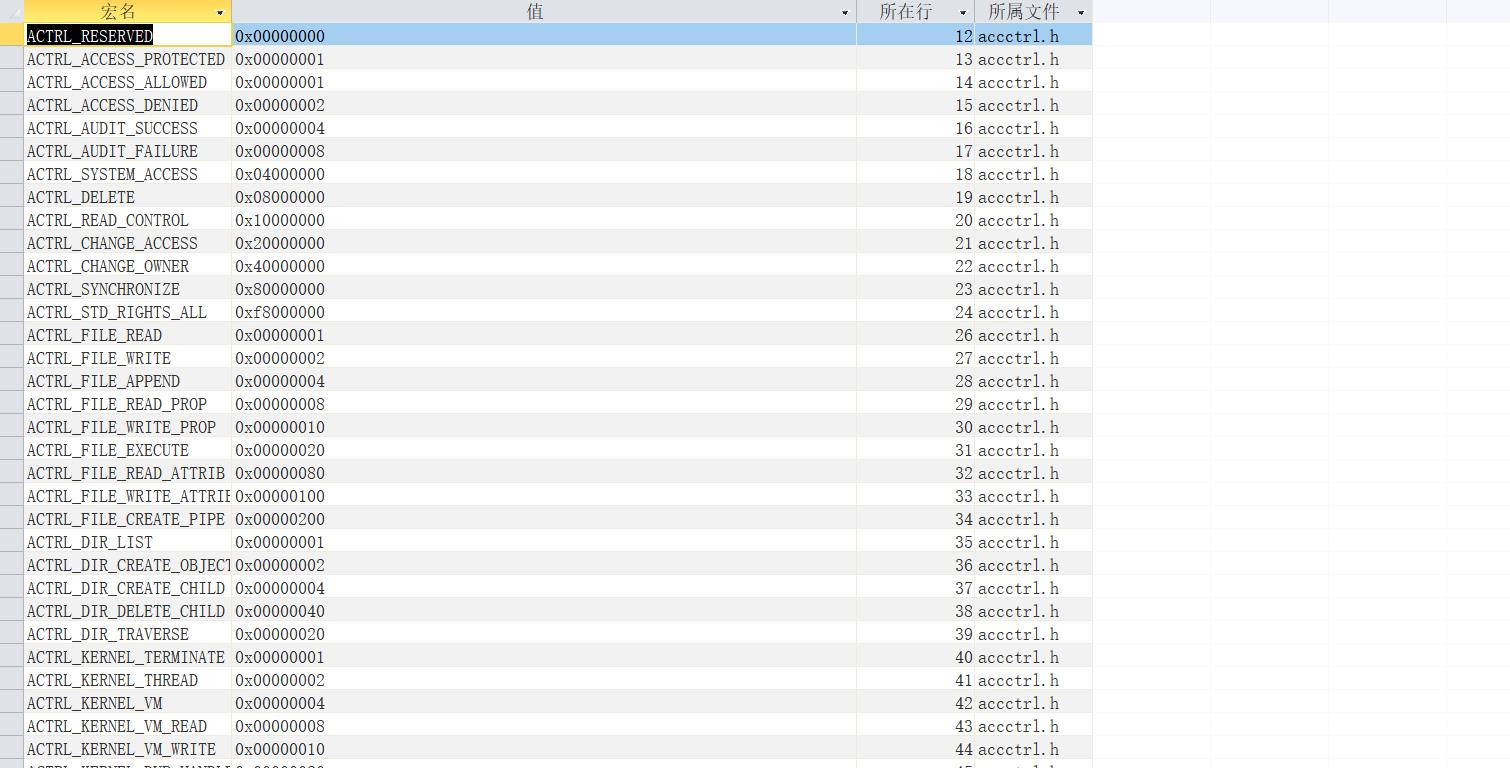•5星
40KB weixin_51194902 2021-01-08 12:19:19
•5星
108KB qq_20232875 2021-01-11 19:16:19
•5星
1.25MB weixin_44573410 2021-03-13 20:36:25
•5星
137KB weixin_44573410 2021-03-02 23:27:18
•5星
56KB qq_40905804 2021-01-27 19:09:08
•5星
1.46MB weixin_44573410 2021-03-15 21:57:51
•5星
435KB weixin_44573410 2021-03-13 20:29:44
•5星
4.4MB I520ZYS 2021-02-19 09:53:22
•5星
712KB weixin_44573410 2021-03-01 13:55:37
•5星
451B qq_24896243 2021-06-15 23:47:28
• C语言函数库 clanguage

1.05MB m0_37384317 2018-09-09 00:12:08
• C语言函数库详解(收藏版) C语言函数库

877KB wjh911103 2018-09-12 11:37:20
• C语言函数库详解 C 函数库

209KB xuer99 2018-04-21 21:41:50
•3星
106KB hualingxin 2010-04-15 16:40:12
• 174KB wwjs314surprise 2016-06-08 15:41:17
• 250KB qq_37340229 2018-07-25 14:25:33
•5星
213KB wolf54 2010-05-09 14:58:13
• C语言函数库和文件 怎么查看c语言库文件

3)把它放在函数库中。下面分别讨论这三种方法。4.6.1程序文件的大小因为C语言允许分别编译，很自然就会提出这样的问题：一个文件的最适宜的规模是多大？这规模很重要，因为编译时间与被编译文件的大小直接相关。...

一个函数设计完后，我们可以用三种方法处理它：1)把它放在main()函数的同一个文件中；2)把它和写好的其它函数一起放在另一个文件中；3)把它放在函数库中。下面分别讨论这三种方法。

4.6.1程序文件的大小

因为C语言允许分别编译，很自然就会提出这样的问题：一个文件的最适宜的规模是多大？这规模很重要，因为编译时间与被编译文件的大小直接相关。一般说来，链接处理的时间比编译处理的时间短得多，且不需要经常去重新编译已经运行过的代码；另一方面，不得

不同时处理多个文件也确实是件厌烦的事。

问题的答案是，每个用户、每个编译程序、每个操作系统环境都是不同的。可是对大部分微型机和一般的C编译程序来说。源程序文件不应长于10000个字节，建立短于5000个字节的文件，可以避免不少麻烦。

4.6.2分类组织文件

在开发一个大型程序时，最令人烦恼的而又是最常遇到的工作之一就是需要检查每个文件，以确定某个函数的存放。在程序开发的早期做一点文件组织工作就可以避免这一问题。

首先可以把概念上有关的函数组织到一个文件中。如果在编写正文编辑程序时，把删除正文所用的所有函数放进另一个文件，等等。

第二，把所有的通用函数放在一起。例如，在数据库程序中，输入／输出格式编排函数是被其它函数调用的通用函数，应把它们放进一个单独的文件里。

第三，把最高层函数放进一个单独的文件中，如果空间允许，就和main()放在一起。最高层函数被用来启动程序的总体活动。这些例程从本质上定义了程序的操作。

4.6.3函数库

从技术上讲，函数库与分别编译的函数文件不同。当库中例程被链接到程序中，或当使用一个分别编译的文件时，文件中的所有函数都被装入和链接到程序中去。对自己创建的函数文件中的大多数文件来说，文件中所有的函数都是要用到的。而对C的标准函数库，永远也无法把所有的函数都连接到自己的程序中去，因为目的码会大得吓人！

有时候我们需要建立一个函数库，例如，假定已经完成了一套专门的统计函数，如果当前开发的某个程序仅仅需要求出一批数值的均值，我们就不必把这些函数全部装入。在这种情况下，函数库是很有用的。

大部分C语言的编译程序都有建立函数库的指令。操作过程因编译程序不同而异，可从用户手册中寻找建库的具体步骤。

展开全文weixin_35792271 2021-05-25 01:50:18
• C语言函数库大全.txt C语言函数库大全

288KB xipengbozai 2021-04-20 23:40:22
• 1.39MB plaugh01 2021-02-04 09:50:18
• 184KB luojiming1990 2012-05-04 21:34:51
• 481KB weixin_38740391 2021-05-14 21:04:02
• C语言函数库 C语言函数库和文件(完).doc c语言 函数

67KB biaozhun100 2011-01-01 03:40:33
• C语言函数库大全.doc c语言

3.8MB qq_32433169 2020-12-04 17:50:29
• 这个主要使用ACCESS来进行制作，可以将C语言函数、全局变量、宏、联合、结构、枚举等类型的字符串分类储存起来，能使用窗体查询这里里面的函数或者宏，也可以用来查看某个函数属于哪个头文件，比如printf()函数： ...

这个主要使用ACCESS来进行制作，可以将C语言的函数、全局变量、宏、联合、结构、枚举等类型的字符串分类储存起来，能使用窗体查询这里里面的函数或者宏，也可以用来查看某个函数属于哪个头文件，比如printf()函数：当然，要是人工一个一个的输函数，还不得累死，因此使用VBA制作了简单的程序，可以从语言的头文件或者源文件自动识别出函数、全局变量、结构、宏、联合、枚举等，然后添加到表中，不过技术能力有限，还有BUG，识别错误，或者报告文件导入失败其实已经成功是常有的事，目前只能作为一个辅助工具来使用。当程序写大了，或者在看不知名的代码，可以使用这个工具导入一下，就可以看到这个文件包含哪些东西。使用效果如图，这里面已经导入了几个C语言标准库的头文件：VB忘很久了，所以代码写的很乱，不算注释空行大概470多行，VBA的代码：

Option Compare Database
Public depth As Integer     '记录当前代码的嵌套深度
Public ann As Boolean       '记录当前代码书否属于多行注释
Public num As Integer       '记录a字符串数组的大小
Public 所属文件 As String   '储存当前正在处理的文件名
Public line As Long         '记录当前所在行
Public sql As String        '储存要执行的DQL语句
Public fnum As Long          '记录函数数量
Public vnum As Long         '记录变量数量
Public model As Integer       '记录当前模式，1：检索标准库，2：导入文件

Label0.Caption = "文件路径:"
command0.Caption = "执行"
model = 2
加入.Visible = False
End Sub

Private Sub 检索标准库_Click()
Label0.Caption = "输入查询的函数或宏："
command0.Caption = "查询"
model = 1

End Sub

Private Sub 加入_Click()
Text0.SetFocus  '将焦点移到文本框，就可以隐藏控件本身
用户确认 = MsgBox("是否加入?", vbYesNo, "warring")
If 用户确认 = vbNo Then GoTo 加入_End
If standlib() Then
MsgBox "导入完成"
Else: MsgBox "导入失败"
End If
加入_End:
加入.Visible = False
End Sub
Private Sub command0_Click()
If model = 1 Then               '判断当前模式，1为查询标准库，2为导入文件
Dim db
Dim 标准库 As Recordset
Dim 找到 As Boolean             '找到函数名或者宏名标志
找到 = False
Set db = CurrentDb              '令db指向当前的数据库
Set 标准库 = db.OpenRecordset("select * from 标准库")   '通过SQL语句获得表
'从text0读取要查询的函数名或宏名
s = Text0.value
If 标准库.EOF Then Exit Sub         '判断当前表是否为空
标准库.MoveFirst                    '将表定位到第一行
Do While Not 标准库.EOF            '遍历表，进行查询
temp = 标准库.Fields("函数名")
If IsNull(temp) Then GoTo l1       '判断该表项是否为空
函数名 = CStr(temp)
If 函数名 = s Then
找到 = True
Text1.value = 函数名
Text2.value = CStr(标准库.Fields("函数参数"))
Text5.value = CStr(标准库.Fields("文件名"))
Text3.value = ""
Text4.value = ""
End If
l1:
标准库.MoveNext         '移动到下一行
Loop
If Not 找到 Then
标准库.MoveFirst
Do While Not 标准库.EOF
On Error GoTo l2
temp = 标准库.Fields("宏名")
If IsNull(temp) Then GoTo l2
宏名 = CStr(temp)
If 宏名 = s Then
找到 = True
Text3.value = 宏名
On Error GoTo nodef
Text4.value = CStr(标准库.Fields("宏定义"))
Text5.value = CStr(标准库.Fields("文件名"))
Text1.value = ""
Text2.value = ""
End If
l2:
标准库.MoveNext
Loop
End If
标准库.Close
If Not 找到 Then
Text1.value = ""
Text2.value = ""
Text3.value = ""
Text5.value = ""
nodef:
Text4.value = ""
End If
Exit Sub
ElseIf model = 2 Then
Dim filename As String
If IsNull(Text0.value) Then Exit Sub
filename = Text0.value
If filename <> "" And filename <> "输入要读取的文件路径" Then filectrl (filename)
model = 2
End If
End Sub

Private Sub Text0_GotFocus()
Text0.Text = ""
End Sub

Private Sub Text0_LostFocus()
If Text0.Text = "" And model <> 1 Then Text0.Text = "输入要读取的文件路径"
End Sub

Private Sub 读取文件_Click()
Label0.Caption = "文件路径:"
command0.Caption = "执行"
model = 2
End Sub

Function filectrl(filename As String) As Boolean
filectrl = False
Dim buf As String       '储存截取出的字符串
Dim strstart As Integer  '记录字符串的起始位置
Dim strend As Integer    '记录字符串的结束位置
Dim i As Integer         '遍历字符串
Dim length As Integer    '记录字符串长度
Dim ann As Boolean       '记录多行注释，当目前内容是注释时，设为真
depth = 0
ann = False
line = 0
is_struct_union_equm = False
fnum = 0
vnum = 0
存在花括号 = False
DoCmd.SetWarnings False      '关掉弹窗警告
'提出文件名
最后一个分割线位置 = InStrRev(filename, "\", Len(filename))
所属文件 = Mid(filename, 最后一个分割线位置 + 1, Len(filename) - 最后一个分割线位置)
On Error GoTo ferr  '如果读取错误，就跳转到ferr这里
Open filename For Input As #1
Do While Not EOF(1) '判断是否到达文件尾
Line Input #1, buf  '读取一行字符串到buf
line = line + 1
'去除注释
buf = subcomment(buf)
If buf = "" Then GoTo donext
buf = Replace(buf, """", """""")
'判断宏
If Mid(buf, 1, 1) = "#" Then
If cdefine(buf) Then GoTo donext
End If
左花括号 = InStr(1, buf, "{")
右花括号 = InStr(1, buf, "}")
If 左花括号 > 0 And 右花括号 = 0 Then 存在花括号 = True
'将结构这样带有定义的关键字整体放到一行
If (InStr(1, buf, "struct") > 0 Or InStr(1, buf, "union") > 0 Or InStr(1, buf, "enum") > 0) And 存在花括号 Then
is_struct_union_equm = True
Do While 存在花括号 And Not EOF(1)
Line Input #1, s
line = line + 1
If InStr(1, s, "}") > 0 Then
存在花括号 = False
buf = buf + s
Else
buf = buf + s
End If
Loop
End If
'判断是否属于结构，联合，枚举等类型
If is_struct_union_equm Then
cblock (buf)
is_struct_union_equm = False '重新置位，防止下一次循环错误使用
GoTo donext
End If
'判断函数
If fun(buf) Then
GoTo donext
'判断变量
ElseIf cvalue(splitStr(buf)) Then
GoTo donext
End If
If 左花括号 > 0 Then depth = depth + 1
If 右花括号 > 0 Then depth = depth - 1
If depth < 0 Then Exit Do
donext:
Loop
Close #1
文件类型 = "未知"
句号未知 = InStr(2, 所属文件, ".")
If 句号位置 > 0 Then
后缀名 = Mid(所属文件, 句号位置, Len(所属文件) - 句号位置)
If 后缀名 = "h" Or 后缀名 = "H" Then
文件类型 = "头文件"
ElseIf 后缀名 = "c" Or 后缀名 = "C" Then
文件类型 = "源文件"
End If
End If
sql = "insert into C代码表(文件名,文件类型,代码行数,函数数量,变量数量,引用到的头文件) values(""" _
+ 所属文件 + """,""" + 文件类型 + """," + str(line) + "," + str(fnum) + "," + str(vnum) + ",""" + headfile + """);"
'MsgBox sql
DoCmd.RunSQL sql
MsgBox "文件导入完成"
加入.Visible = True
filectrl = True
Exit Function
ferr:   '文件读取错误
Close #1
MsgBox "文件路径错误"
End Function

'函数处理
Function fun(buf)
Dim 右 As Boolean
右 = False
fun = False
'查找括号，如果存在括号，则将括号前以非英文字符、数字、下划线划分的字符串提出
length = InStr(1, buf, "(")
左花括号 = InStr(1, buf, "{")
右花括号 = InStr(1, buf, "}")
等于号 = InStr(1, buf, "=")
If 等于号 <> 0 Then GoTo exitfun
If length = 0 Then GoTo exitfun

If 左花括号 > 0 And 左花括号 < length Then
depth = depth + 1
Exit Function
End If
If 右花括号 > 0 And 右花括号 < length Then
depth = depth - 1
右 = True
End If
strend = length
If strend > 0 And depth = 0 Then  '存在括号且嵌套深度为0
a = splitStr(buf)
函数类型 = ""
For i = 1 To num
t = InStr(1, a(i), "(")
If t > 0 Then
If t > 1 Then
函数名 = Mid(a(i), 1, t - 1)
For j = 1 To i - 1
函数类型 = 函数类型 + a(j) + " "
Next
Else
函数名 = a(i - 1)
For j = 1 To i - 2
函数类型 = 函数类型 + a(j) + " "
Next
End If
Exit For
End If
Next
'取出函数参数
t = InStr(length, buf, ")")
If t <> 0 Then
函数参数 = Mid(buf, length, t - length + 1)
'MsgBox "函数参数：" + 函数参数
End If
End If
If 函数名 <> "" Then
fun = True
Else
Exit Function
End If
sql = "insert into 函数(函数名,类型,参数,所在行,所属文件,引用到的函数,定义) values(""" + _
函数名 + """,""" + 函数类型 + """,""" + 函数参数 + """," + str(line) + ",""" + 所属文件 + _
""",""" + "未知" + """,""" + "未知" + """);"
'MsgBox sql
DoCmd.RunSQL sql
fnum = fnum + 1
exitfun:
If 左花括号 > 0 Then depth = depth + 1
If 右花括号 > 0 And Not 右 Then depth = depth - 1
End Function

'去除注释
Function subcomment(buf)
Dim length As Integer
Dim strstart As Integer
Dim strend As Integer
strstart = 1
strend = -1
'将所有换行符替换为空格
length = InStr(1, buf, "*/")
If length > 0 Then
ann = False
strstart = length + 2  'i指向有效的字符串位置
End If

If ann Then
subcomment = ""
Exit Function
End If

If strstart > Len(buf) Then
subcomment = ""
Exit Function
End If

length = InStr(strstart, buf, "/*")
If length > 0 Then
strend = length - 1
ann = True
End If
length = InStr(strstart, buf, "//")
If length > 0 Then
strend = length - 1
End If
If strend = -1 Then
strend = Len(buf)
ElseIf strend = 0 Then
subcomment = ""
Exit Function
End If
'通过strstart和strend两个下标值确定非注释字符串的位置，取出字符串
buf = Mid(buf, strstart, strend - strstart + 1)
'去除字符串左右两边的多余空格，制表符
buf = Replace(buf, vbTab, " ") '将制表符全部替换为空格
buf = Trim(buf)
subcomment = buf
End Function
'宏处理
Function cdefine(buf)
cdefine = False
第一个空格的位置 = InStr(1, buf, " ")
If 第一个空格的位置 < 2 Then
Exit Function
End If
第一个字符串 = Mid(buf, 2, 第一个空格的位置 - 2)
If 第一个字符串 = "define" Then
第二个空格的位置 = InStr((第一个空格的位置 + 1), buf, " ")
length = 0
If 第二个空格的位置 = 0 Then
If Len(buf) > 第一个空格的位置 Then
length = Len(buf) - 第一个空格的位置
Else
Exit Function
End If
Else
length = 第二个空格的位置 - 第一个空格的位置 - 1
End If
第二个字符串 = Mid(buf, 第一个空格的位置 + 1, length)
Else
Exit Function
End If
If 第二个空格的位置 > 0 Then
第三个字符串 = Mid(buf, 第二个空格的位置, Len(buf) + 1 - 第二个空格的位置)
Else
第三个字符串 = ""
End If
'MsgBox "宏：" + 第一个字符串
'MsgBox "宏名：" + 第二个字符串
'MsgBox "宏定义：" + 第三个字符串
sql = "insert into 宏(宏名,值,所在行,所属文件) values(""" + Trim(第二个字符串) + """,""" + Trim(第三个字符串) + """," + str(line) + ",""" + 所属文件 + """);"
'MsgBox sql
DoCmd.RunSQL sql
cdefine = True
End Function
'全局变量处理，函数内部的变量识别复杂，而且通常情况没有必要，因此没有实现
Function cvalue(a)
Dim i As Integer
cvalue = False
类型 = ""
变量名 = ""
值 = ""
If depth <> 0 Then
Exit Function
End If
'遍历a，查看是否有=，如果有说明该变量被赋值
找到等于号 = False
For i = 1 To num
等于号位置 = InStr(1, a(i), "=")
If 等于号位置 > 0 Then
找到等于号 = True
Exit For
End If
Next
If 找到等于号 Then
For j = 1 To i - 2
类型 = 类型 + a(j) + " "
Next
If 等于号位置 = 1 Then '说明该a(i)第一个字符为等于号
变量名 = a(i - 1)
值 = a(num)

Else
变量名 = Mid(a(i), 1, 等于号位置 - 1)
类型 = 类型 + a(i - 1) + " "
值 = Mid(a(i), 等于号位置 + 1, Len(a(i)) - 等于号位置)
End If
Else
变量名 = a(num)
For j = 1 To num - 1
类型 = 类型 + a(j) + " "
Next
End If
'MsgBox 变量名
'MsgBox 类型
'MsgBox 值
cvalue = True
sql = "insert into 变量(变量名,类型,初始值,所在行,所属文件) values(""" + 变量名 + """,""" + 类型 + """,""" + 值 + """," + str(line) + ",""" + 所属文件 + """);"
DoCmd.RunSQL sql
vnum = vnum + 1
End Function

'按空格分割字符串，返回一个字符串数组，这个数组的长度由全集变量num说明
Function splitStr(codestr)
Dim a() As String
num = 1
Dim i As Integer
i = 1
Do While i < Len(codestr)
b = InStr(i, codestr, " ")
If b <> 0 Then
If b > i Then
ReDim Preserve a(num)
a(num) = Trim(Mid(codestr, i, b - i))
num = num + 1
End If
i = b + 1
Else
Exit Do
End If
Loop
'还有最后一个字符串可能没有读入
ReDim Preserve a(num)
a(num) = Trim(Mid(codestr, i, Len(codestr) - i))
splitStr = a
End Function

'对结构，联合，枚举的处理
Function cblock(buf)
cblock = False
左花括号 = InStr(1, buf, "{")
右花括号 = InStr(1, buf, "}")
If 左花括号 = 0 Or 右花括号 = 0 Then
Exit Function
End If
's储存关键字名
s = Trim(Mid(buf, 1, 左花括号 - 1))
定义 = Trim(Mid(buf, 左花括号, 右花括号 - 左花括号 + 1))
别名 = Trim(Mid(buf, 右花括号 + 1, Len(buf) - 右花括号 - 1))
If InStr(1, s, "struct") > 0 Then
sql = "insert into 结构(结构名,定义,别名,所在行,所属文件) values(""" + s + """,""" + 定义 + """,""" + 别名 + """," + str(line) + ",""" + 所属文件 + """);"
DoCmd.RunSQL sql
ElseIf InStr(1, s, "union") > 0 Then
sql = "insert into 联合体(联合体名,定义,别名,所在行,所属文件) values(""" + s + """,""" + 定义 + """,""" + 别名 + """," + str(line) + ",""" + 所属文件 + """);"
DoCmd.RunSQL sql
ElseIf InStr(1, s, "enum") > 0 Then
sql = "insert into 枚举(枚举名,定义,别名,所在行,所属文件) values(""" + s + """,""" + 定义 + """,""" + 别名 + """," + str(line) + ",""" + 所属文件 + """);"
DoCmd.RunSQL sql
Else
Exit Function
End If
'MsgBox "名：" + s
'MsgBox "定义：" + 定义
'MsgBox "别名：" + 别名
cblock = True
End Function
'处理头文件
inc = Mid(buf, 2, 7)
If inc = "include" Then
'读取后面括号或者引号之间的文件名
左 = InStr(9, buf, "<")
If 左 > 0 Then
右 = InStr(10, buf, ">")
If 右 = 0 Then
Exit Function
End If
headfile = headfile + Mid(buf, 左, 右 - 左 + 1) + ";"
Else
左 = InStr(9, buf, """")
右 = InStr(10, buf, """")
If 左 = 0 Or 右 = 0 Then
Exit Function
End If
headfile = headfile + Mid(buf, 左, 右 - 左 + 1) + ";"
End If
End If
End Function

'标准库操作，将函数表和宏表合并到标准库表
Function standlib() As Boolean
standlib = False
函数表可用 = True
宏表可用 = True
Dim db
Dim 宏表 As Recordset
Dim 函数表 As Recordset
Dim s As String       '储存读取标识符
Set db = CurrentDb    '设定为当前数据库
Set 宏表 = db.OpenRecordset("select * from 宏")
Set 函数表 = db.OpenRecordset("select * from 函数")
If 宏表.EOF And 函数表.EOF Then Exit Function

If 宏表.EOF Then
宏表可用 = False
Else: 宏表.MoveFirst
End If
If 函数表.EOF Then
函数表可用 = False
Else: 函数表.MoveFirst
End If

Do While (Not 宏表.EOF) Or (Not 函数表.EOF)
If Not 宏表.EOF And 宏表可用 Then
On Error GoTo fnext              '错误处理，一般情况是读取到了空表项，此时利用goto来跳转
宏名 = CStr(宏表.Fields("宏名"))
On Error GoTo fnext
宏定义 = Replace(CStr(宏表.Fields("值")), """", """""")
On Error GoTo fnext
文件名 = CStr(宏表.Fields("所属文件"))
sql = "insert into 标准库(宏名,宏定义,文件名) values(""" + 宏名 + """,""" _
+ 宏定义 + """,""" + 文件名 + """);"
On Error GoTo fnext
DoCmd.RunSQL sql
standlib = True
fnext:
宏表.MoveNext
End If
If Not 函数表.EOF And 函数表可用 Then
On Error GoTo anext
函数名 = CStr(函数表.Fields("函数名"))
On Error GoTo anext
函数参数 = CStr(函数表.Fields("参数"))
On Error GoTo anext
文件名 = CStr(函数表.Fields("所属文件"))
sql = "insert into 标准库(函数名,函数参数,文件名) values(""" + 函数名 + """,""" _
+ 函数参数 + """,""" + 文件名 + """);"
On Error GoTo anext
DoCmd.RunSQL sql
standlib = True
anext:
函数表.MoveNext
End If
Loop
宏表.Close      '关闭表
函数表.Close
End Function

展开全文lindorx 2019-07-04 10:51:42
• 1.85MB feixiangdeyanzi123 2021-10-26 14:35:34
• C语言函数库大全 C语言函数库大全

1.1MB s1377427321 2012-06-26 07:39:12
• 366KB luojiming1990 2012-03-30 17:04:59
• 481KB weixin_38654382 2021-05-20 17:06:29
• 17KB weixin_38617196 2021-05-11 18:21:19
• csv表格操作C语言函数库 csv C语言5星
20KB tianfeirj 2009-09-04 12:17:45
•4星
633KB wangwenwen 2011-11-09 00:27:39
• c语言函数库 c 函数

214KB hao630077468 2011-12-02 23:21:51
• C语言函数库[参照].pdf 软件开发

155KB xhr131452007 2021-10-11 01:38:44...

c语言函数库c语言 订阅